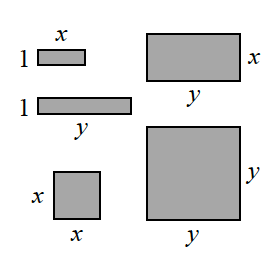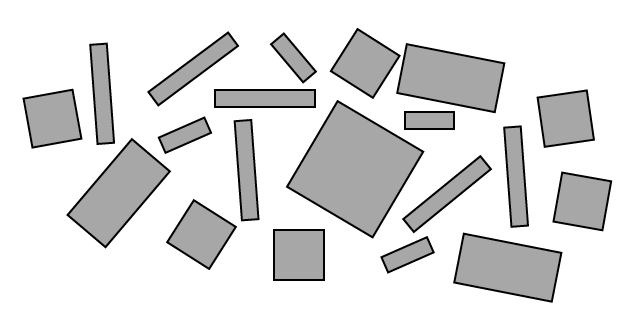Home > ACC7 > Chapter cc32 > Lesson cc32.1.2 > Problem2-18

2-18.If the tiles have the dimensions shown at right, what is the name of the tile collection below? (That is, what is the total area of all of the pieces?) Write the expression algebraically, using $x$, $x^2$, $y$, $y^2$, and $xy$. 2-18 HW eTool (CPM). Homework Help ✎To solve this problem, you will need to group the shapes by their area. How many $x$-tiles are there? $x^2$-tiles?
Count the number of each different type of tile.

$6x^2+4x+3xy+6y+y^2$

Use the tiles in the eTool below to write the algebraic expression.
Click the link at right for the full version of the eTool: CC3 2-18 HW eTool